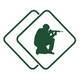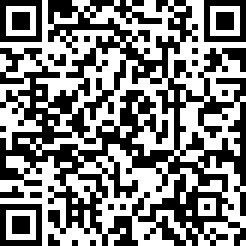#### ASVAB Armed Services Vocational Aptitude Battery (Exam 27)

created by Fisher BRink (@fisher) at Feb. 8, 2016
• An audience consists of M people, and 2/3 of the audience are adults. C the adults...

• A rectangular vegetable garden 16 yards long and 4 yards wide is completely enclos...

• What is the maximum number of boxes, each measuring 3 inches by 4 inches by 5 inch...

• A circle passes through the four vertices of a rectangle that is 8 feet long and 6...

• There are 12 liters of a mixture of acetone in alcohol that is 33 % acetone. 1 lo...

• If x = 8, what's the value of y in the equationy = (x2 ÷ 4) 2?

• The cube of 5 is:

• 5 × 33 =

• The fourth root of 16 is:

• What's the equation of a line that passes through points (0, -1) and (2, 3)?

• (12 yards + 14 feet) ÷ 5 =

• x3 × x4 =

• (x + 4)(x + 2) =

• 5 × 103 =

• Which of the following is a prime number?

• What's the mode of the following series of numbers? 4 4 8 8 8 10 10 12 12

• If a = 4, then a3 ÷ a =

• Solve for the factorial of 5 (5!):

• What is the value of (0.2)3?

• If x2 + x = 6, what is the value of x?

Be the first to review

• info
Quiz Info
• date_range
Feb. 8, 2016, 3:26 a.m.
help_outline
20 questions
dvr
0 completed
remove_red_eye
13 views
people
0 takers
folder•#### Ratings

star_borderstar_borderstar_borderstar_borderstar_border
ratings